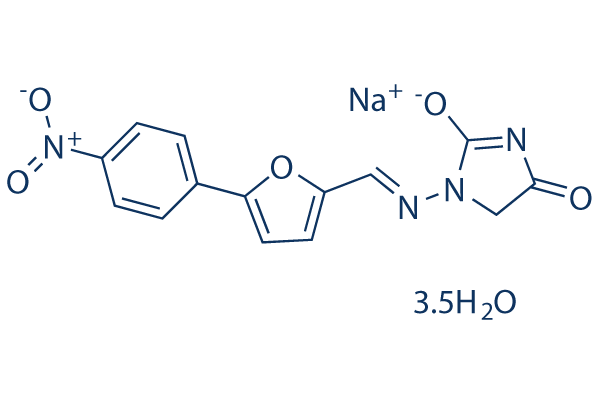# Dantrolene sodium hemiheptahydrate

For research use only.

Catalog No.S4648 Synonyms: Dantrolene sodium salt, Dantrium, Sodium dantrolene

2 publicationsCAS No. 24868-20-0

Dantrolene sodium hemiheptahydrate (Dantrium) ia a skeletal muscle relaxant that acts by interfering with excitation-contraction coupling in the muscle fiber.

### Biological Activity

Description Dantrolene sodium hemiheptahydrate (Dantrium) ia a skeletal muscle relaxant that acts by interfering with excitation-contraction coupling in the muscle fiber. Dantrolene sodium is a skeletal muscle relaxant, which inhibits intracellular Ca2+ release from the sarcoplasmic reticulum. Dantrolene sodium shows strong antioxidant activity in the linoleic acid emulsion system. Dantrolene sodium (5 mg/kg) has a greater depressant action on the twitch contraction of the fast extensor digitorum longus (EDL) muscle than on the slow soleus (SOL) muscle.

### Protocol

Animal Research: - Collapse Animal Models: Sprague Dawley rats Dosages: 5 mg/kg Administration: i.v.(Only for Reference)

### Solubility (25°C)

 In vitro DMSO 14 mg/mL (35.06 mM) Water Insoluble Ethanol Insoluble

* Please note that Selleck tests the solubility of all compounds in-house, and the actual solubility may differ slightly from published values. This is normal and is due to slight batch-to-batch variations.

Molecular Weight 399.29 C14H9N4O5.7/2H2O.Na 24868-20-0 3 years-20°C powder 2 years-80°C in solvent Dantrolene sodium salt, Dantrium, Sodium dantrolene C1C(=NC(=O)N1N=CC2=CC=C(O2)C3=CC=C(C=C3)[N+](=O)[O-])[O-].C1C(=NC(=O)N1N=CC2=CC=C(O2)C3=CC=C(C=C3)[N+](=O)[O-])[O-].O.O.O.O.O.O.O.[Na+].[Na+]

### In vivo Formulation Calculator (Clear solution)

 Step 1: Enter information below (Recommended: An additional animal making an allowance for loss during the experiment) Dosage mg/kgAverage weight of animals gDosing volume per animal ulNumber of animals Step 2: Enter the in vivo formulation (This is only the calculator, not formulation. Please contact us first if there is no in vivo formulation at the solubility Section.) % DMSO+ % PEG300 Corn oil + % Tween 80+ % ddH2O CalculateReset

### Bio Calculators

Molarity Calculator

## Molarity Calculator

Calculate the mass, volume or concentration required for a solution. The Selleck molarity calculator is based on the following equation:

### Mass (mg) = Concentration (mM) × Volume (mL) × Molecular Weight (g/mol)

• Mass
Concentration
Volume
Molecular Weight

*When preparing stock solutions, please always use the batch-specific molecular weight of the product found on the via label and SDS / COA (available on product pages).

Dilution Calculator

## Dilution Calculator

Calculate the dilution required to prepare a stock solution. The Selleck dilution calculator is based on the following equation:

### Concentration (start) x Volume (start) = Concentration (final) x Volume (final)

This equation is commonly abbreviated as: C1V1 = C2V2 ( Input Output )

• C1
V1
C2
V2

* When preparing stock solutions always use the batch-specific molecular weight of the product found on the vial label and SDS / COA (available online).

## The Serial Dilution Calculator Equation

• ### Computed Result

• C1=C0/X C2=C1/X C1: LOG(C1): C2: LOG(C2): C3: LOG(C3): C4: LOG(C4): C5: LOG(C5): C6: LOG(C6): C7: LOG(C7): C8: LOG(C8):
Molecular Weight Calculator

## Molecular Weight Calculator

Enter the chemical formula of a compound to calculate its molar mass and elemental composition:

### Total Molecular Weight: g/mol

Tip: Chemical formula is case sensitive. C10H16N2O2 c10h16n2o2

#### Instructions to calculate molar mass (molecular weight) of a chemical compound:

To calculate molar mass of a chemical compound, please enter its chemical formula and click 'Calculate'.

#### Definitions of molecular mass, molecular weight, molar mass and molar weight:

Molecular mass (molecular weight) is the mass of one molecule of a substance and is expressed in the unified atomic mass units (u). (1 u is equal to 1/12 the mass of one atom of carbon-12)
Molar mass (molar weight) is the mass of one mole of a substance and is expressed in g/mol.

#### Molarity Calculator

 Mass Concentration Volume Molecular Weight pg ng μg mg g kg = fM pM nM μM mM M × nL μL mL L ×

### Tech Support

Answers to questions you may have can be found in the inhibitor handling instructions. Topics include how to prepare stock solutions, how to store inhibitors, and issues that need special attention for cell-based assays and animal experiments.

Tel: +1-832-582-8158 Ext:3